# NCERT Solutions for Class 7 Maths Chapter 6 The Triangle and its Properties Ex 6.5

NCERT Solutions for Class 7 Maths Chapter 6 The Triangle and its Properties Ex 6.5

### NCERT Solutions for Class 7 Maths Chapter 6 The Triangle and its Properties Ex 6.5

NCERT Solutions for Class 7 Maths Chapter 6 The Triangle and its Properties Exercise 6.5
Ex 6.5 Class 7 Maths Question 1.
PQR is a triangle, right angled at P. If PQ = 10 cm and PR = 24 cm, find QR.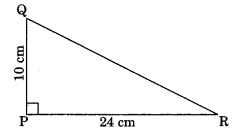Solution:
In right angled triangle PQR, we have
QR2 = PQ2 + PR2 From Pythagoras property)
= (10)2 + (24)2
= 100 + 576 = 676The, the required length of QR = 26 cm.

Ex 6.5 Class 7 Maths Question 2.
ABC is a triangle, right angled at C. If AB = 25 cm and AC = 7 cm, find BC.Solution:
In right angled∆ABC, we have
BC2 + (7)2 = (25)2 (By Pythagoras property)
⇒ BC2 + 49 = 625
⇒ BC2 = 625 – 49
⇒ BC2 = 576Thus, the required length of BC = 24 cm.

Ex 6.5 Class 7 Maths Question 3.
A 15 m long ladder reached a window 12 m high from the ground on placing it against a wall at a distance a. Find the distance of the foot of the ladder from the wall.Solution:
Here, the ladder forms a right angled triangle.
∴ a2 + (12)2 = (15)2 (By Pythagoras property)
⇒ a2+ 144 = 225
⇒ a2 = 225 – 144
⇒ a2 = 81Thus, the distance of the foot from the ladder = 9m

Ex 6.5 Class 7 Maths Question 4.
Which of the following can be the sides of a right triangle?
(i) 2.5 cm, 6.5 cm, 6 cm.
(ii) 2 cm, 2 cm, 5 cm.
(iii) 1.5 cm, 2 cm, 2.5 cm
Solution:
(i) Given sides are 2.5 cm, 6.5 cm, 6 cm.
Square of the longer side = (6.5)2 = 42.25 cm.
Sum of the square of other two sides
= (2.5)2 + (6)2 = 6.25 + 36
= 42.25 cm.
Since, the square of the longer side in a triangle is equal to the sum of the squares of other two sides.
∴ The given sides form a right triangle.

(ii) Given sides are 2 cm, 2 cm, 5 cm .
Square of the longer side = (5)2 = 25 cm Sum of the square of other two sides
= (2)2 + (2)2 =4 + 4 = 8 cm
Since 25 cm ≠ 8 cm
∴ The given sides do not form a right triangle.

(iii) Given sides are 1.5 cm, 2 cm, 2.5 cm
Square of the longer side = (2.5)2 = 6.25 cm Sum of the square of other two sides
= (1.5)2 + (2)2 = 2.25 + 4
Since 6.25 cm = 6.25 cm = 6.25 cm
Since the square of longer side in a triangle is equal to the sum of square of other two sides.
∴ The given sides form a right triangle.

Ex 6.5 Class 7 Maths Question 5.
A tree is broken at a height of 5 m from the ground and its top touches the ground at a distance of 12 m from the base of the tree . Find the original height of the tree.
Solution:
Let AB be the original height of the tree and broken at C touching the ground at D such that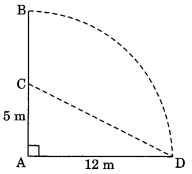AC = 5 m
AD2 + AC2 = CD2 (By Pythagoras property)
⇒ (12)2 + (5)2 = CD2
⇒ 144 + 25 = CD2
⇒ 169 = CD2But CD = BC
AC + CB = AB
5 m + 13 m = AB
∴ AB = 18 m .
Thus, the original height of the tree = 18 m.

Ex 6.5 Class 7 Maths Question 6.
Angles Q and R of a APQR are 25° and 65°. Write which of the following is true.
(i) PQ2 + QR2 = RP2
(ii) PQ2 + RP2 = QR2
(iii) RP2 + QR2 = PQ2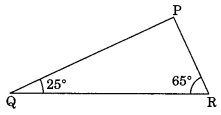Solution:
We know that
∠P + ∠Q + ∠R = 180° (Angle sum property)
∠P + 25° + 65° = 180°
∠P + 90° = 180°
∠P = 180° – 90° – 90°
∆PQR is a right triangle, right angled at P
(i) Not True
∴ PQ2 + QR2 ≠ RP2 (By Pythagoras property)
(ii) True
∴ PQ2 + RP2 = QP2 (By Pythagoras property)
(iii) Not True
∴ RP2 + QR2 ≠ PQ2 (By Pythagoras property)

Ex 6.5 Class 7 Maths Question 7.
Find the perimeter of the rectangle whose length is 40 cm and a diagonal is 41 cm.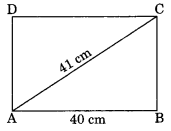Solution:
Given: Length AB = 40 cm
Diagonal AC = 41 cm
In right triangle ABC, we have
AB2 + BC2= AC2 (By Pythagoras property)
⇒ (40)2 + BC2 = (41)2
⇒ 1600 + BC2 = 1681
⇒ BC2 = 1681 – 1600
⇒ BC2 = 81∴ AB = DC = 40 cm and BC = AD = 9 cm (Property of rectangle)
∴ The required perimeter
= AB + BC + CD + DA
= (40 + 9 + 40 + 9) cm
= 98 cm

Ex 6.5 Class 7 Maths Question 8.
The diagonals of a rhombus measure 16 cm and 30 cm. Find its perimeter.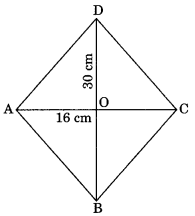Solution:
Let ABCD be a rhombus whose diagonals intersect each other at O such that AC = 16 cm and BD = 30 cm
Since, the diagonals of a rhombus bisect each other at 90°.
∴ OA = OC = 8 cm and OB = OD = 15 cm
In right ∆OAB,
AB2 = OA2 + OB2 (By Pythagoras property)
= (8)2+ (15)2 = 64 + 225
= 289Since AB = BC = CD = DA (Property of rhombus)
∴ Required perimeter of rhombus
= 4 × side = 4 × 17 = 68 cm.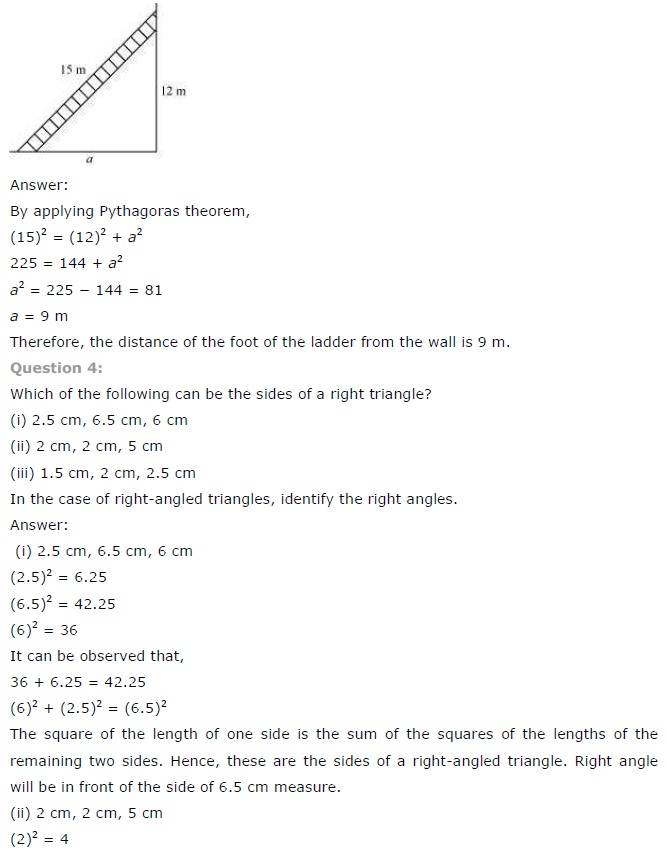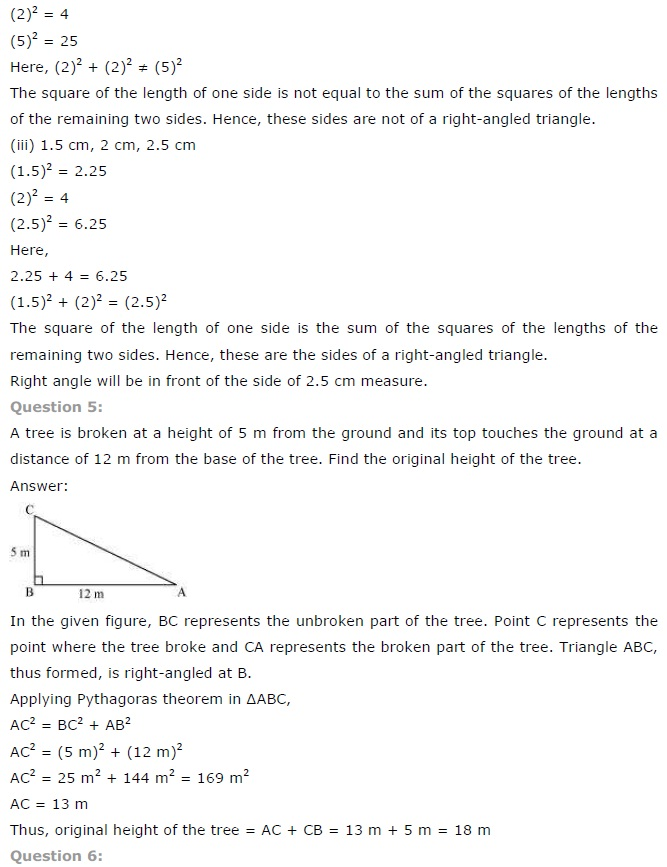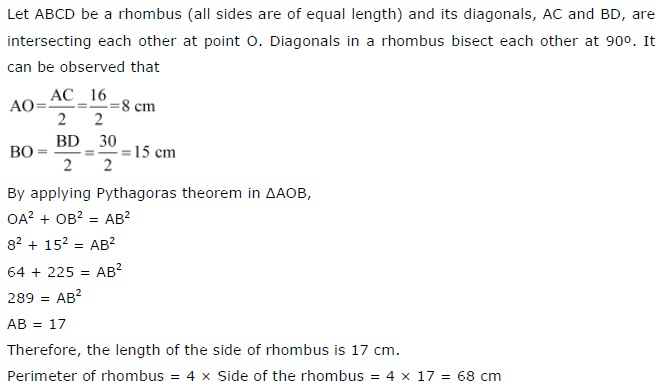## SabDekho

The Complete Educational Website